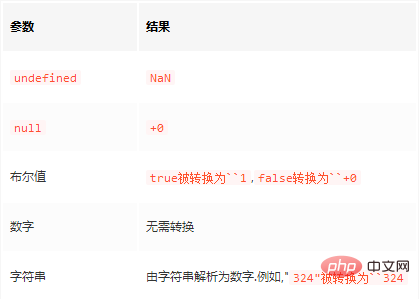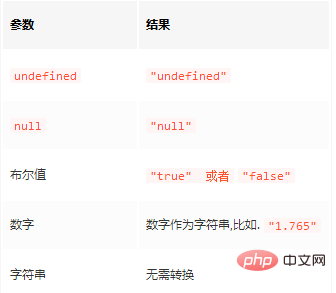JavaScript类型转换（详解及实例）JavaScript 加法规则

• 数字和数字相加

• 字符串和字符串相加

• 原始值有：undefined、null、 布尔值(boolean)、 数字(number)、字符串(string)、symbol

• 对象值：其他的所有值都是对象类型的值，包括数组(arrays)和函数(functions)

ToPrimitive(input, PreferredType?)

• 如果输入的值已经是个原始值,则直接返回它.

• 否则,如果输入的值是一个对象.则调用该对象的valueOf()方法.如果valueOf()方法的返回值是一个原始值,则返回这个原始值.

• 否则,调用这个对象的toString()方法.如果toString()方法的返回值是一个原始值,则返回这个原始值.

• 否则,抛出TypeError异常.demo

var obj = {
valueOf: function () {
console.log("valueOf");
return {}; // 没有返回原始值
},
toString: function () {
console.log("toString");
return {}; // 没有返回原始值
}
}

Number作为一个函数被调用(而不是作为构造函数调用)时,会在引擎内部调用ToNumber()操作:

Number(obj)
// output
valueOf
toString
Uncaught TypeError: Cannot convert object to primitive value
String(obj)
// output
toString
valueOf
Uncaught TypeError: Cannot convert object to primitive value

value1 + value2

• 将两个操作数转换为原始值 (下面是数学表示法,不是JavaScript代码):

prim1 := ToPrimitive(value1)
prim2 := ToPrimitive(value2)

PreferredType被省略,因此 Date 类型的值采用String,其他类型的值采用Number.

• 如果 prim1 或者 prim2 中的任意一个为字符串，则将另外一个也转换成字符串，然后返回两个字符串连接操作后的结果；

• 否则，将 prim1 和 prim2 都转换为数字类型，返回他们的和。

[]+[]

[]会被转换成一个原始值,首先尝试 valueOf() 方法,返回数组本身(this):

> var arr = [];
> arr.valueOf() === arr
true

> [] + {}
'[object Object]'

{} + {}

• JavaScript引擎将第一个{}解释成了一个空的代码块并忽略了它

• 这里的加号并不是代表加法的二元运算符，而是一个一元运算符，作用是将它后面的操作数转换成数字，和 Number() 函数完全一样。例如:

+{}
Number({})
Number({}.toString())  // 因为{}.valueOf()不是原始值
Number("[object Object]")
NaN
> {} + []
0
• {} 忽略

• +[] = Number([]) = Number([].toString()) = Number("") = 0

> {} + {}
'[object Object][object Object]'
> {} + []
'[object Object]'

Javacript 中 + 号工作：

• 数字 + 数字

• 字符串 + 字符串

【相关推荐：javascript学习教程

全部评论我要评论

• 取消发布评论发送
• 1/1